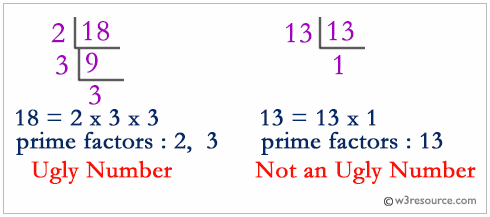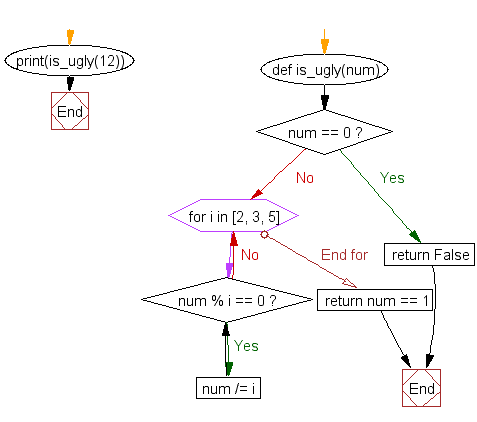﻿ Python: Check whether a given number is an ugly number - w3resource

# Python Challenges: Check whether a given number is an ugly number

## Python Challenges - 1: Exercise-24 with Solution

Write a Python program to check whether a given number is an ugly number.

Ugly numbers are positive numbers whose only prime factors are 2, 3 or 5. The sequence 1, 2, 3, 4, 5, 6, 8, 9, 10, 12, ...
shows the first 10 ugly numbers.
Note: 1 is typically treated as an ugly number.

Explanation:Sample Solution:

Python Code:

``````def is_ugly(num):
if num == 0:
return False
for i in [2, 3, 5]:
while num % i == 0:
num /= i
return num == 1

print(is_ugly(12))
print(is_ugly(13))
```
```

Sample Output:

```True
False
```

Flowchart:Python Code Editor: